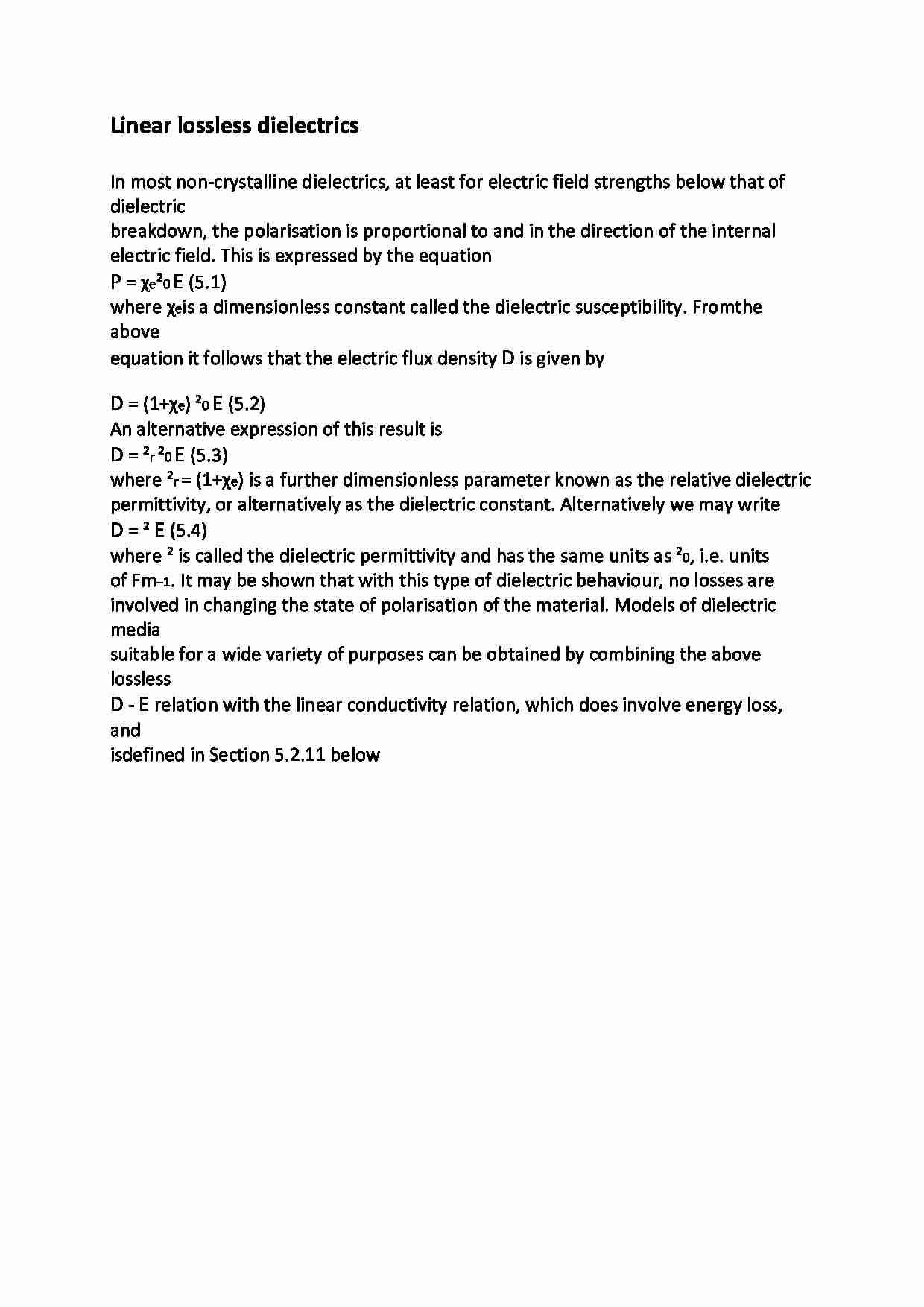# Linear lossless dielectrics - wykład

Nasza ocena:

Wyświetleń: 567
Komentarze: 0

### Pobierz ten dokument za darmo

Podgląd dokumentu## Fragment notatki:

Linear lossless dielectrics In most non-crystalline dielectrics, at least for electric field strengths below that of dielectric
breakdown, the polarisation is proportional to and in the direction of the internal
electric field. This is expressed by the equation
P = χe ²0 E (5.1)
where χe is a dimensionless constant called the dielectric susceptibility. Fromthe above
equation it follows that the electric flux density D is given by
D = (1+χe) ²0 E (5.2)
An alternative expression of this result is
D = ²r ²0 E (5.3)
where ²r = (1+χe) is a further dimensionless parameter known as the relative dielectric
permittivity, or alternatively as the dielectric constant. Alternatively we may write
D = ² E (5.4)
where ² is called the dielectric permittivity and has the same units as ²0, i.e. units
of Fm−1. It may be shown that with this type of dielectric behaviour, no losses are
involved in changing the state of polarisation of the material. Models of dielectric media
suitable for a wide variety of purposes can be obtained by combining the above lossless
D - E relation with the linear conductivity relation, which does involve energy loss, and
is defined in Section 5.2.11 below
... zobacz całą notatkę

## Komentarze użytkowników (0)

Zaloguj się, aby dodać komentarz